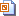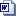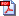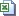Nuffield Mathematics teaching resources are for use in secondary and further education

# Level 2 Data handling

Students use official data about accidents to calculate relative frequencies and probabilities of different accidents occurring. Emphasis is given to the reliability both of the data and the conclusions that can be drawn.Slides (1.3 MB)Student sheets WordStudent sheets pdfTeacher notes WordTeacher notes pdf

## Sports injuries

Students consider the difficulties in using real data to answer questions such as ‘Which is the most dangerous sport? Students discuss which types of statistical diagram are most appropriate for displaying the data before setting off to draw them. They may practise drawing statistical diagrams either using Excel or by hand.

## Larks and owls

A very open investigation in which students may set their own statistical tasks based on sleep requirements.

## Music Festival

Students use weather data to consider which month would be the best to hold an outdoor music festival. They practise using either a calculator in Statistics mode or a spreadsheet to calculate mean and standard deviation values.

## Currency conversion

An introduction to conversion graphs and direct proportionality in the context of currency conversion, reading and plotting graphs and calculating gradients. One question uses a spreadsheet.Slides (1.4 MB)Student sheets Word 2007 (74 KB)Student sheets pdf (444 KB)Teacher notes Word (78 KB)Teacher notes pdf (457 KB)

## Pay rates for men and women - Excel activity

Students are shown how to draw and format cumulative frequency graphs in Excel.Spreadsheet (720 KB)Student sheets for Excel 2007 Word (2.3 MB)Student sheets for Excel 2007 pdf (1.0 MB)Teacher notes Word (93 KB)Teacher notes pdf (404 KB)

## Part-time work survey

A statistical investigation into students’ paid employment. Students design questionnaires, find averages and the range, and draw charts and graphs. Data analysis can be on paper or spreadsheets.Slides (1.5 MB)Student sheets Word (243 KB)Student sheets pdf (505 KB)Teacher notes Word (438 KB)Teacher notes pdf (76 KB)

## Plumber's call-out

Students complete data tables, then draw, read and interpret linear graphs where the intercept on the y axis is not zero. Interpretation includes finding where two linear graphs meet. The last part requires a spreadsheet – omit if computers not available. For level 2 the work could be refocused to introduce algebraic graphs.

## Shorter by helicopter

Students plot graphs of real data to compare the straight-line distances between towns with the distance by road.

## Five a day

Students collect data and then use statistical methods to analyse the results.

## Heart rate

Students are shown how to measure their pulse rate. They then investigate the effect that other things like exercise and relaxation have on it.Slides (1.1 MB)Student sheets Word (159 KB)Student sheets pdf (319 KB)Teacher notes Word (140 KB)Teacher notes pdf (357 KB)